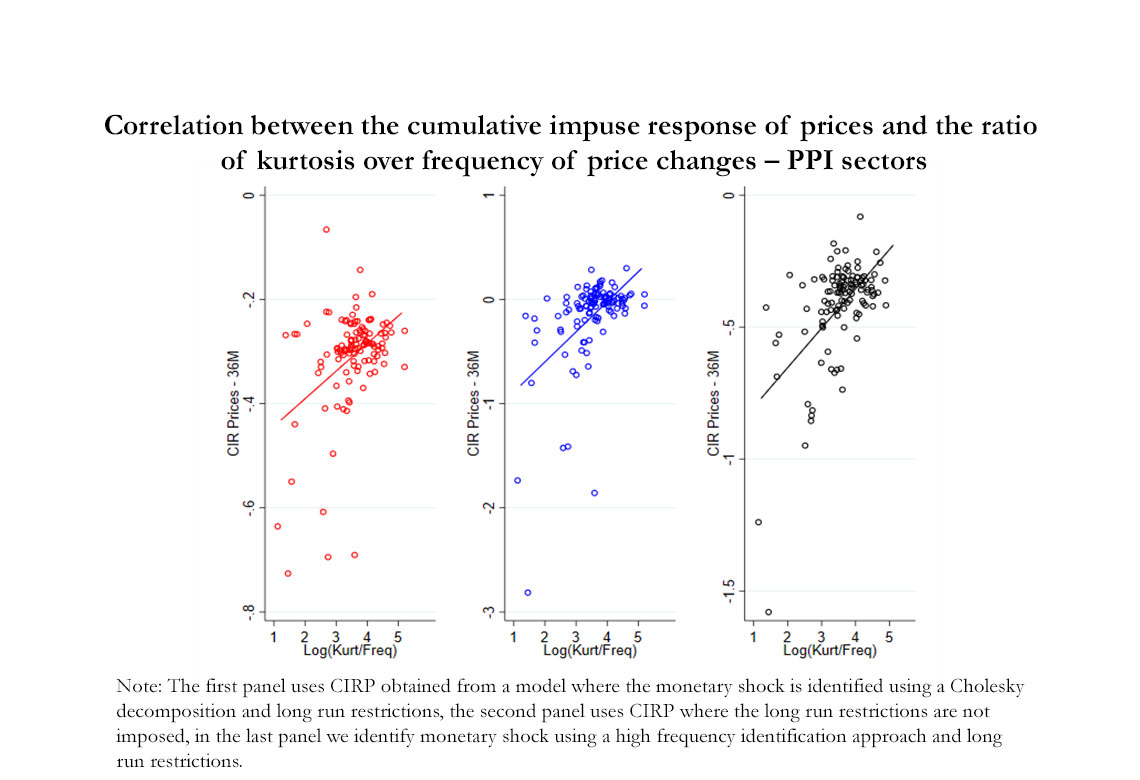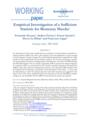# Working Paper Series no. 839: Empirical Investigation of a Sufficient Statistic for Monetary Shocks

In a broad class of sticky price models the non-neutrality of nominal shocks is encoded by a simple sufficient statistic: the ratio of the kurtosis of the size-distribution of price changes over the frequency of price changes. We test this theoretical prediction using data for a large number of firms representative of the French economy. We use the micro data to measure the cross sectional moments, including kurtosis and frequency, for about 120 PPI industries and 220 CPI categories. We use a Factor Augmented VAR to measure the sectoral responses to a monetary shock, as summarized by the cumulative impulse response of sectoral prices (CIRP), under three alternative identification schemes. The estimated CIRP correlates with the kurtosis and the frequency consistently with the prediction of the theory - i.e. they enter the relationship as a ratio. The analysis also shows that other moments not suggested by the theory, such as the mean, standard deviation and skewness of the size-distribution of price changes, are not correlated with the CIRP. Several robustness checks are explored.In the area of monetary economics, recent theoretical results have identified a “sufficient statistic” for monetary shocks for a broad class of sticky-price models under low inflation. The key proposition is that the cumulative response of output to a once-and-for-all small monetary shock - essentially the area under the output impulse response - is proportional to the ratio of two cross-sectional statistics of the price change distribution. These two statistics are the kurtosis of the steady-state distribution of (non-zero) price changes (a measure of “fat tails” in this distribution) and the frequency of price changes.

An intuition of why these two moments of the price change distribution do capture the effects of monetary shocks is the following. If price changes are infrequent, prices will respond slowly to a shock, inducing larger real effects. So the inverse of the frequency of price changes captures the overall degree of price rigidity, a feature which is standard in most sticky price models. For a given frequency of price changes, the patterns of the size of price adjustments also matter. In particular, if firms adjusting their prices are the ones whose prices are very far from the price that would have prevailed under price flexibility, the price response will be larger and quicker, and the output effect smaller. The kurtosis of price changes turns out to capture this “price selection” effect. More price selection is associated to a lower kurtosis, to a faster response of the aggregate price to a shock and to lower the real effects of monetary shocks. This price selection effect is maximal in a standard menu cost model and minimal in a standad Calvo model.

This paper presents an empirical test of the predictions of the sufficient-statistic proposition using the restrictions implied by the theory for an economy with low inflation. The theoretical prediction is somewhat bold: the ratio of kurtosis to frequency should explain different degrees of monetary non neutrality, while other moments should not matter (e.g. the variance, or the skewness, of price changes). We proceed to testing the sufficient-statistic proposition using producer price indices (PPI) and consumer price indices (CPI) micro data for a large number of firms that are representative of the French economy. Our approach to perform the test has three steps. First, we estimate the sectoral responses to a monetary shock for about 120 PPI industries and 220 CPI categories, using a Factor Augmented VAR (FAVAR) model. The second step consists in using the micro data underlying the sectoral data to measure the cross sectional moments of the distribution of price changes (frequency, kurtosis of price changes) in the different sectors. In the third step, we inspect the relationship between the CIRP and the cross-sectoral moments under the restrictions implied by the theory.

The results consistently show that the data do not reject the predictions of the theory across a variety of tests, specifications, and robustness exercises. In particular, Figure A illustrates the positive correlation across PPI sectors between the ratio of kurtosis over frequency and the cumulative impulse response of prices. Each panel is a scatter plot of CIRP  over the log-ratio for three different specifications of monetary policy shocks. Using OLS regressions, we show that these correlations are significant. Moreover, we show that both the frequency and the kurtosis appear as statistically significant factors in accounting for the cross-sectional heterogeneity of the estimated CIRP for both the PPI data, as well for the CPI data. The sign and magnitudes of the estimated coefficients are consistent with the predictions of the theory in the specification where the variables enter the regression as a ratio. An unrestricted specification where both variables are entered as separate regressors also provides results consistent with theory. Moreover, “placebo” tests show that moments not suggested by the theory, such as the size, standard deviation and skewness of price changes, are not correlated with the CIRP.